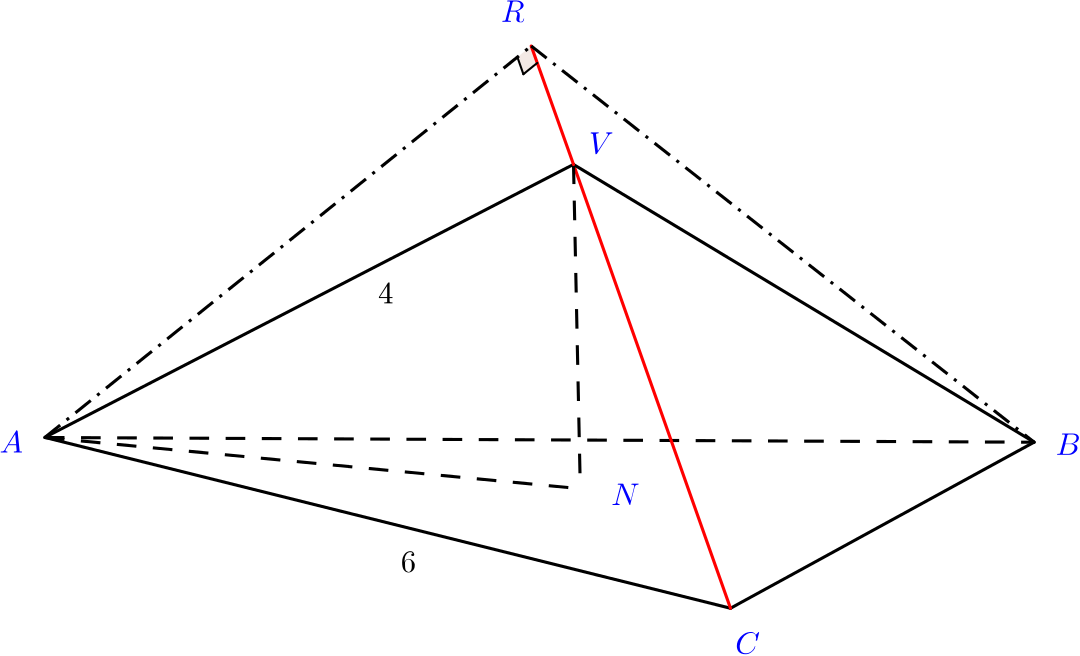Review question

# Can we find the lengths and angles in this pyramid? Add to your resource collection Remove from your resource collection Add notes to this resource View your notes for this resource

Ref: R6589

## Solution

An equilateral triangle $ABC$ has side length $6$ units. The three altitudes of the triangle meet at $N$. Show that $AN=2\sqrt{3}$ units.

To find the length $AN$, let’s call the midpoint of $AC, M$, and the midpoint of $BC, P$.

Then the triangles $ANM$ and $ACP$ are similar, since they are both right-angled, and they also share another angle.

Using Pythagoras, we find that $AP=\sqrt{6^2-3^2}=\sqrt{36-9}=\sqrt{27}=3\sqrt{3}$. We know that

$\frac{AN}{3}=\frac{AN}{AM}=\frac{AC}{AP}=\frac{6}{3\sqrt{3}},$

since the triangles are similar, and so $AN=\dfrac{6}{\sqrt{3}}=6\dfrac{\sqrt{3}}{3}=2\sqrt{3}$ units.

Alternatively, we could have recognised that $ANM$ is a standard $30$-$60$-$90$ triangle with edges in the ratio $1:2:\sqrt{3}$.

This triangle is the base of a pyramid whose apex $V$ lies on the line through $N$ perpendicular to the plane $ABC$. Given that $VN=2$ units, prove that $\widehat{VAN}=30^\circ.$

We know $AN$, and $VN$ is given in the question giving $\tan\widehat{VAN}=\dfrac{2}{2\sqrt 3}=\dfrac{1}{\sqrt 3}$ which we should recognize as $\tan 30^\circ$ giving $\widehat{VAN}=30^\circ.$

Alternatively we find $AV = 4$, by Pythagoras.

We now have that $\sin\widehat{VAN}=\frac{2}{4}=\frac{1}{2},$ Therefore $\widehat{VAN}=30^\circ.$

The perpendicular from $A$ to the edge $VC$ meets $CV$ produced at $R$. Prove that $AR=\dfrac{3}{2}\sqrt{7}$ units, and find the exact value of $\cos \widehat{ARB}$.The triangle $AVC$

By symmetry, we know that $AV=VC$. We’ll use the cosine rule to find $\widehat{ACV}$.

Applying the cosine rule to $ACV$, we have that \begin{align*} 4^2&=6^2+4^2-2\times 4\times 6\times \cos\widehat{ACV}, \\ 48\cos\widehat{ACV}&=36, \\ \cos\widehat{ACV}&=\frac{3}{4}. \end{align*}

Now by right-angled trigonometry, we have that $\sin\widehat{ACV}=\frac{AR}{6},$ and so $AR=6\sin\widehat{ACV}=6\sqrt{1-\cos^2\widehat{ACV}}=6\sqrt{\frac{7}{16}}=\frac{6}{4}\sqrt{7}=\frac{3}{2}\sqrt{7}.$

Alternatively, we could say $RV = x$, and $AR = y$, and then use Pythagoras twice to create simultaneous equations that enable us to find $y$.

We need now to compute $\cos\widehat{ARB}$.

By symmetry, we have that $BR=AR$. So we can apply the cosine rule to $ARB$.The triangle $ARB$ We have that \begin{align*} 6^2&=2\left(\frac{3\sqrt{7}}{2}\right)^2-2\left(\frac{3\sqrt{7}}{2}\right)\left(\frac{3\sqrt{7}}{2}\right)\cos\widehat{ARB}, \\ \implies 36&=\frac{9\times 7}{2}-\frac{9\times 7}{2}\cos\widehat{ARB} \\ \implies 63\cos\widehat{ARB}&=63-72,\\ \implies \cos\widehat{ARB}&=-\frac{9}{63}=-\frac{1}{7}. \end{align*}